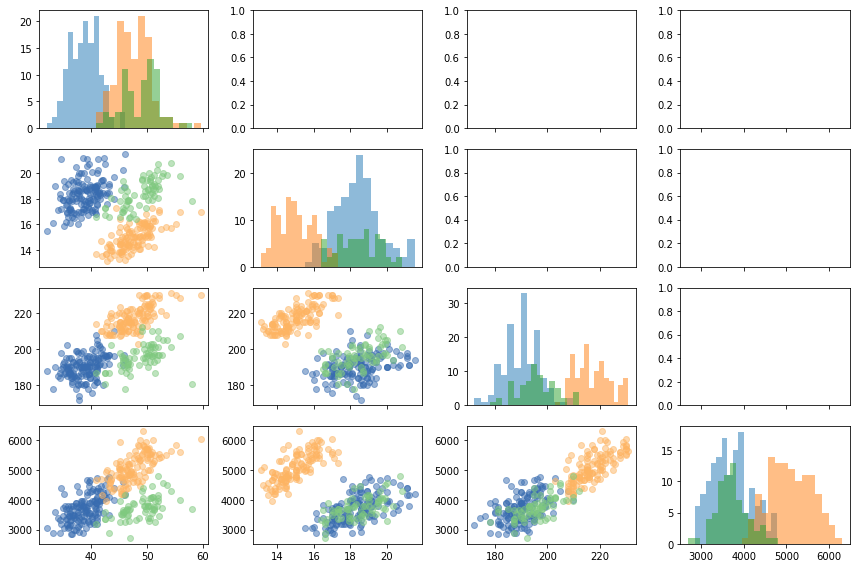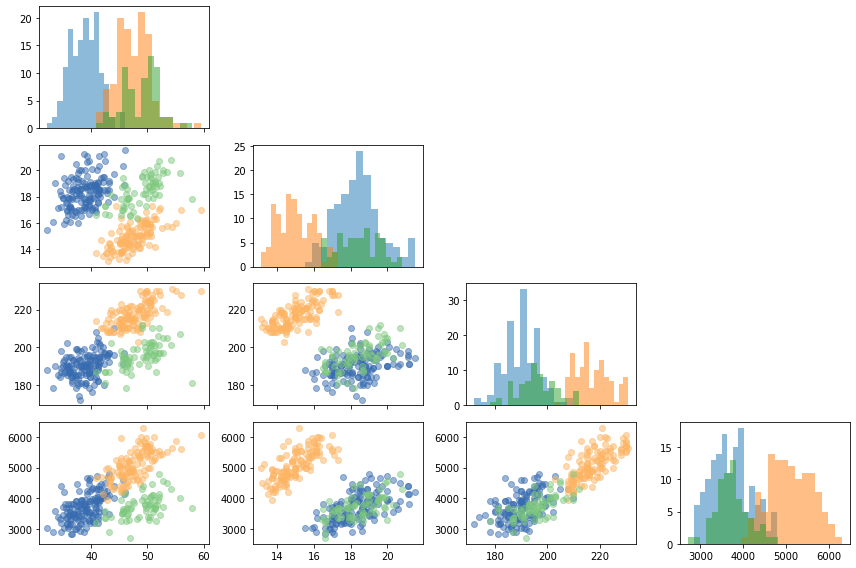# How to remove axis in Matplotlib

The aim of this post is to show you how to remove certain axis from a vizualisation that is made up of multiple subplots. To do so, we build a pairplot or correlogram from scratch and show how to remove empty axis from the final chart.

``````import palmerpenguins
import matplotlib.pyplot as plt``````
``````penguins = palmerpenguins.load_penguins().dropna()
species island bill_length_mm bill_depth_mm flipper_length_mm body_mass_g sex year
0 Adelie Torgersen 39.1 18.7 181.0 3750.0 male 2007
1 Adelie Torgersen 39.5 17.4 186.0 3800.0 female 2007
2 Adelie Torgersen 40.3 18.0 195.0 3250.0 female 2007
4 Adelie Torgersen 36.7 19.3 193.0 3450.0 female 2007
5 Adelie Torgersen 39.3 20.6 190.0 3650.0 male 2007

## Pairplot with Matplotlib

Let's create a pairplot with Matplotlib from scratch. This chart is useful to see the relationship between numerical variables and their distributions for some groups of observations. In this case, observations are grouped according to the species. The numerical variables are those in the `COLUMNS` list.

``````COLUMNS = ["bill_length_mm", "bill_depth_mm", "flipper_length_mm", "body_mass_g"]
COLORS = ["#386cb0", "#fdb462", "#7fc97f"]

And now the plot:

``````# A layout of 4x4 subplots
fig, axes = plt.subplots(4, 4, figsize = (12, 8), sharex="col", tight_layout=True)

for i in range(len(COLUMNS)):
for j in range(len(COLUMNS)):
# If this is the lower-triangule, add a scatterlpot for each group.
if i > j:
for species, color in zip(SPECIES, COLORS):
data = penguins[penguins["species"] == species]
axes[i, j].scatter(COLUMNS[j], COLUMNS[i], color=color, alpha=0.5, data=data)

# If this is the main diagonal, add histograms
if i == j:
for species, color in zip(SPECIES, COLORS):
data = penguins[penguins["species"] == species]
axes[i, j].hist(COLUMNS[j], bins=15, alpha=0.5, data=data)``````## Remove unused axes

The code above shows it is not that hard to create a pairplot from scratch with Matplotlib. But those empty subplots on the upper triangle are now something we would like to keep on our viz. Let's remove them!

`ax.remove()` is all we need. It simply removes the axis from the figure. Let's loop through the axes again, but this time removing those on the upper triangle of the layout.

``````for i in range(len(COLUMNS)):
for j in range(len(COLUMNS)):
# If on the upper triangle
if i < j:
axes[i, j].remove()

# See the chart now
fig``````## Contact & Edit

👋 This document is a work by Yan Holtz. Any feedback is highly encouraged. You can fill an issue on Github, drop me a message onTwitter, or send an email pasting `yan.holtz.data` with `gmail.com`.